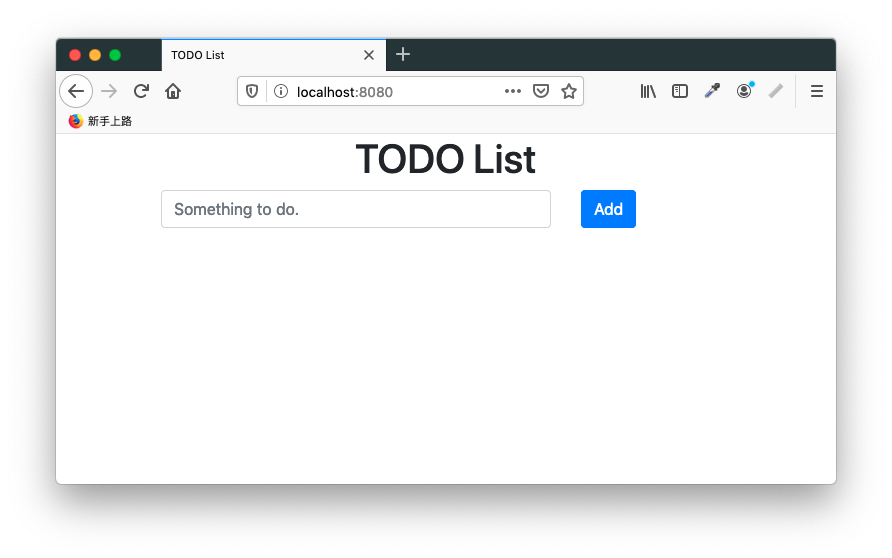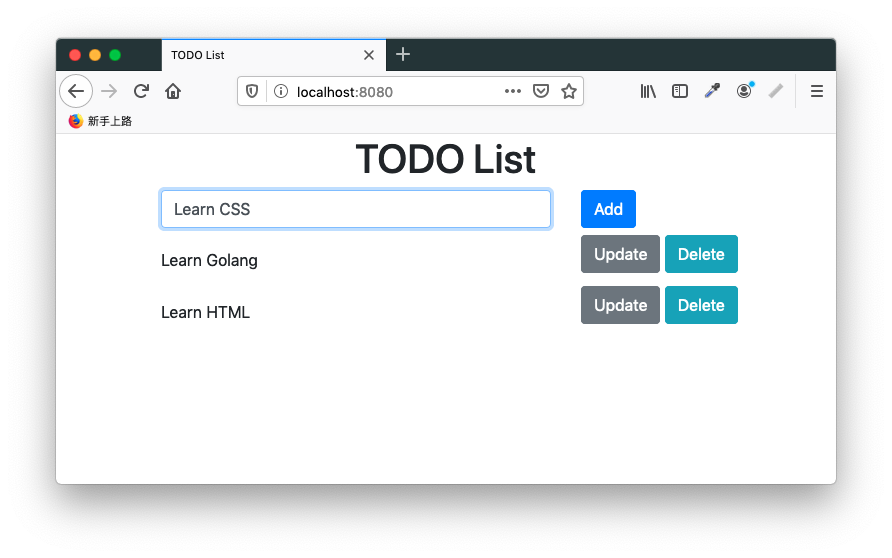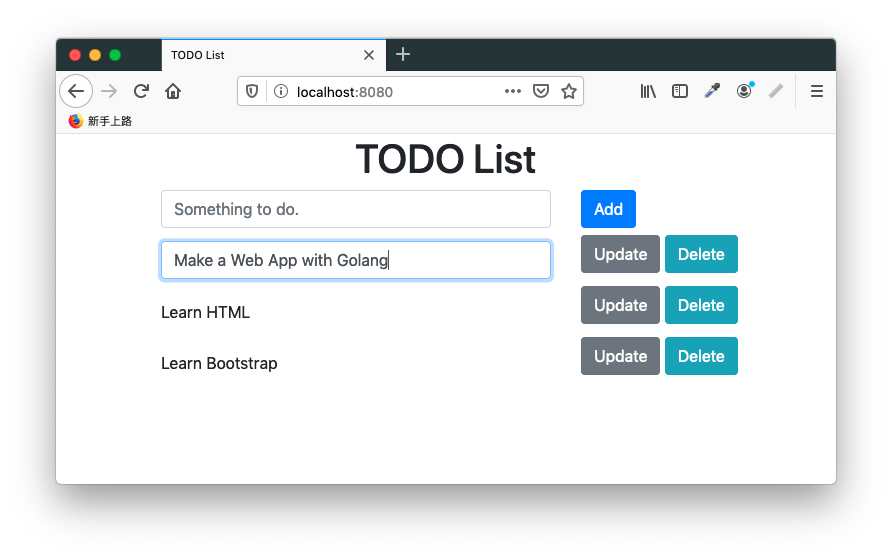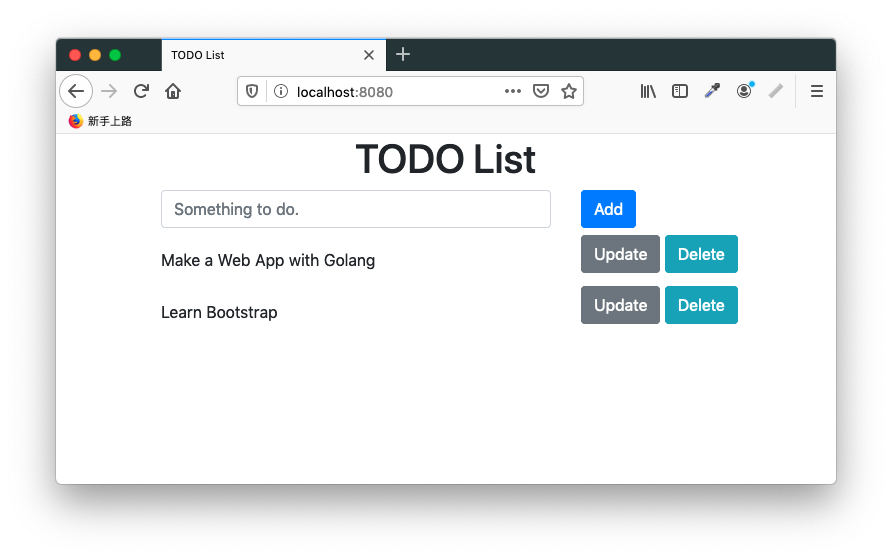#[Golang] 網頁設計敎學：撰寫 HTML 表單 (Form)

## 展示本範例程式## 起始畫面

``mux.GET("/", indexHandler)``

``````func indexHandler(
w http.ResponseWriter,
r *http.Request,
p httprouter.Params) {
var tmpl = template.Must(
template.ParseFiles(
"views/layout.html",
"views/index.html",
)

var msg string

// Clean current message.
}

rows, err := db.Table("todos").Select("*").Rows()
if err != nil {
msg = "Unable to retrieve database"
}

var todos []TODO

todos = make([]TODO, 0)

for rows.Next() {
var todo struct {
ID   uint
Todo string `gorm:"todo"`
}

db.ScanRows(rows, &todo)

todos = append(todos, TODO{
Index: todo.ID,
Item:  todo.Todo,
})
}

data := struct {
Title   string
TODOs   []TODO
Message string
}{
Title:   "TODO List",
TODOs:   todos,
Message: msg,
}

err = tmpl.ExecuteTemplate(w, "layout", data)
if err != nil {
http.Error(w, err.Error(), 500)
}
}``````

``````rows, err := db.Table("todos").Select("*").Rows()
if err != nil {
msg = "Unable to retrieve database"
}

var todos []TODO
todos = make([]TODO, 0)

for rows.Next() {
var todo struct {
ID   uint
Todo string `gorm:"todo"`
}

db.ScanRows(rows, &todo)

todos = append(todos, TODO{
Index: todo.ID,
Item:  todo.Todo,
})
}``````

`db.Table("todos").Select("*").Rows()` 會回傳資料庫對表格 `todos` 查詢 (query) 的結果，這時資料庫游標 (cursor) 會停留在查詢結果的第一行 (record)。我們接著以 `rows.Next()` 逐一走訪查詢結果的每一行。以 `db.ScanRows(rows, &todo)` 將該行的資料掃出來。

``````for (let i = 0; i < todos.length; i++) {
for (let j = 0; j < todos.length; j++) {
let label = todos[j].querySelector('label');

if (i === j) {
if (label) {
let text = label.innerText;

let input = document.createElement('input');

input.name = 'todo';
input.value = text;

let index = todos[j].querySelector('[name="index"]').getAttribute('value');

let inputIndex = document.createElement('input');

inputIndex.setAttribute('value', index);
inputIndex.name = 'index'
inputIndex.setAttribute('hidden', true);

todos[i].innerHTML = '';
todos[i].appendChild(input);
todos[i].appendChild(inputIndex);
}
} else {
if (!label) {
let input = todos[j].querySelector('input');

let text = input.value;

let label = document.createElement('label');

label.innerText = text;

let index = todos[j].querySelector('[name="index"]').getAttribute('value');

let inputIndex = document.createElement('input');

inputIndex.setAttribute('value', index);
inputIndex.name = 'index'
inputIndex.setAttribute('hidden', true);

todos[j].innerHTML = '';
todos[j].appendChild(label);
todos[j].appendChild(inputIndex);
}
}
}
});
}``````

``````document.addEventListener('keydown', function (event) {
if (event.which === 27) {
for (let i = 0; i < todos.length; i++) {
let label = todos[i].querySelector('label');

if (!label) {
let input = todos[i].querySelector('input');

let text = input.value;

let label = document.createElement('label');

label.innerText = text;

let index = todos[i].querySelector('[name="index"]').getAttribute('value');

let inputIndex = document.createElement('input');

inputIndex.setAttribute('value', index);
inputIndex.name = 'index'
inputIndex.setAttribute('hidden', true);

todos[i].innerHTML = '';
todos[i].appendChild(label);
todos[i].appendChild(inputIndex);
}
}
}
})``````

## 新增 TODO 項目

``````<form action='/todo/' method='POST'>
<div>
<div>
<input type='text' name='todo' placeholder='Something to do.'>
</div>

<div>
</div>
</div>
</form>``````

``mux.POST("/todo/", updateTODOHandler)``

``````func updateTODOHandler(
w http.ResponseWriter,
r *http.Request,
p httprouter.Params) {
r.ParseForm()

todo := r.FormValue("todo")
method := r.FormValue("_method")

if todo == "" {
} else if method == "update" {
// Implement it later.
} else if method == "delete" {
// Implement it later.
} else {
db.Table("todos").Create(struct {
Todo string `gorm:"todo"`
}{
Todo: todo,
})
}

http.Redirect(w, r, "/", http.StatusSeeOther)
}``````

`todo` 為空時，我們會在網頁 header 中寫入錯誤訊息。若 `todo` 確實有值，我們將其寫入資料庫：

``````db.Table("todos").Create(struct {
Todo string `gorm:"todo"`
}{
Todo: todo,
})``````

``http.Redirect(w, r, "/", http.StatusSeeOther)``

## 更新 TODO 項目

``````{{ range \$t := .TODOs }}
<form action='/todo/' method='POST'>
<div>
<div class='todo'>
<label>{{ \$t.Item }}</label>
<inupt name='index' name='index' value='{{ \$t.Index }}' hidden>
</div>

<div>
<button type='submit' name='_method' value='update' onclick="updateTODO(event);">Update</button>
<button type='submit' name='_method' value='delete' onclick="deleteTODO(event);">Delete</button>
</div>
</div>
</form>
{{ end }}``````

``````<button type='submit' name='_method' value='update' onclick="updateTODO(event);">Update</button>
<button type='submit' name='_method' value='delete' onclick="deleteTODO(event);">Delete</button>``````

``````function updateTODO (event) {
let form = event.target.parentNode.parentNode.parentNode;

let todo = form.querySelector('.todo');

let label = todo.querySelector('label');

if (label) {
let text = label.innerText;

let input = document.createElement('input');

input.name = 'todo';
input.value = text;

let index = todo.querySelector('[name="index"]').getAttribute('value');

console.log(todo.querySelector('[name="index"]'));
console.log(`index: \${index}`);

let inputIndex = document.createElement('input');

inputIndex.setAttribute('value', index);
inputIndex.name = 'index'
inputIndex.setAttribute('hidden', true);

todo.innerHTML = '';
todo.appendChild(input);
todo.appendChild(inputIndex);
}

form.setAttribute('_method', 'update');
form.submit();
}``````

``form.setAttribute('_method', 'update');``

``````func updateTODOHandler(
w http.ResponseWriter,
r *http.Request,
p httprouter.Params) {
r.ParseForm()

todo := r.FormValue("todo")
method := r.FormValue("_method")

if todo == "" {
} else if method == "update" {
index := r.FormValue("index")

if index == "" {
r.Header.Set("Message", "Unable to retrieve TODO item")
} else {
db.Table("todos").Where("id == ?", index).Update(struct {
Todo string `gorm:"todo"`
}{
Todo: todo,
})
}
} else if method == "delete" {
// Implement it later.
} else {
// Create a TODO item.
}

http.Redirect(w, r, "/", http.StatusSeeOther)
}``````

## 刪除 TODO 項目

``````{{ range \$t := .TODOs }}
<form action='/todo/' method='POST'>
<div>
<div class='todo'>
<label>{{ \$t.Item }}</label>
<inupt name='index' name='index' value='{{ \$t.Index }}' hidden>
</div>

<div>
<button type='submit' name='_method' value='update' onclick="updateTODO(event);">Update</button>
<button type='submit' name='_method' value='delete' onclick="deleteTODO(event);">Delete</button>
</div>
</div>
</form>
{{ end }}``````

``<button type='submit' name='_method' value='delete' onclick="deleteTODO(event);">Delete</button>``

``````function deleteTODO (event) {
let form = event.target.parentNode.parentNode.parentNode;

let todo = form.querySelector('.todo');

let label = todo.querySelector('label');

if (label) {
let text = label.innerText;

let input = document.createElement('input');

input.name = 'todo';
input.value = text;

let index = todo.querySelector('[name="index"]').getAttribute('value');

console.log(todo.querySelector('[name="index"]'));
console.log(`index: \${index}`);

let inputIndex = document.createElement('input');

inputIndex.setAttribute('value', index);
inputIndex.name = 'index'
inputIndex.setAttribute('hidden', true);

todo.innerHTML = '';
todo.appendChild(input);
todo.appendChild(inputIndex);
}

form.setAttribute('_method', 'delete');
form.submit();
}``````

``````func updateTODOHandler(w http.ResponseWriter, r *http.Request, p httprouter.Params) {
r.ParseForm()

todo := r.FormValue("todo")
method := r.FormValue("_method")

if todo == "" {
} else if method == "update" {
// Update a TODO item.
} else if method == "delete" {
index := r.FormValue("index")

if index == "" {
r.Header.Set("Message", "Unable to retrieve TODO item")
} else {
db.Table("todos").Where("id == ?", index).Delete(struct {
ID   uint
Todo string
}{})
}
} else {
// Create a TODO item.
}

http.Redirect(w, r, "/", http.StatusSeeOther)
}``````